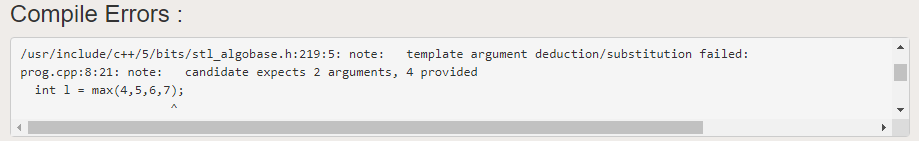# Ellipsis in C++ with Examples

• Last Updated : 19 Aug, 2020

Ellipsis in C++ allows the function to accept an indeterminate number of arguments. It is also known as the variable argument list. Ellipsis tells the compiler to not check the type and number of parameters the function should accept which allows the user to pass the variable argument list. By default, functions can only take a fixed number of parameters that are known to the function beforehand. The user cannot pass a variable number of arguments.

Program 1:

Want to learn from the best curated videos and practice problems, check out the C++ Foundation Course for Basic to Advanced C++ and C++ STL Course for foundation plus STL.  To complete your preparation from learning a language to DS Algo and many more,  please refer Complete Interview Preparation Course.

Below is the code that gives compilation error:

## C++

 `// C++ program to demonstrate the``// compilation error as max function``// doesn't take more than 2 arguments``#include ``using` `namespace` `std;`` ` `// Driver Code``int` `main()``{``    ``// Below Line will given Compilation``    ``// Error as 4 arguments are passed``    ``// instead of 2``    ``int` `l = max(4, 5, 6, 7);``    ``cout << l;``    ``return` `0;``}`

Output:
Below is the output of the above program:Explanation:

max() function can take only 2 arguments. So when 4 arguments were used to it in the function call, the compiler threw an error. However, there may be some situations a function has to take a variable number of arguments.

We can pass number of arguments using Ellipsis. It is defined under the cstdarg header file. Ellipsis is not a keyword rather it is denoted by ‘…’ sign.

Below is the program to illustrate the use of Ellipsis:

## C++

 `// C++ program to demonstrate the``// use of Ellipsis``#include ``#include ``using` `namespace` `std;`` ` `// Function accepting variable number``// of arguments using Ellipsis``double` `average(``int` `count, ...)``{``    ``// va_list found in and``    ``// list is its type, used to``    ``// iterate on ellipsis``    ``va_list` `list;`` ` `    ``// Initialize position of va_list``    ``va_start``(list, count);`` ` `    ``double` `avg = 0.0;`` ` `    ``// Iterate through every argument``    ``for` `(``int` `i = 0; i < count; i++) {``        ``avg += ``static_cast``<``double``>(``va_arg``(list, ``int``))``               ``/ count;``    ``}`` ` `    ``// Ends the use of va_list``    ``va_end``(list);`` ` `    ``// Return the average``    ``return` `avg;``}`` ` `// Driver Code``int` `main()``{``    ``// Function call``    ``double` `avg = average(6, 1, 2, 3, 4, 5, 6);`` ` `    ``// Print Average``    ``cout << ``"Average is "` `<< avg;``    ``return` `0;``}`
Output:
```Average is 3.5
```

Explanation:

Here, average() takes six arguments and calculates the average. Let us see how it works.

• va_list type is used to access the values in the ellipsis. It will be conceptually easy for you if you think of ellipsis as an array. In that case, va_list  will act as the iterator type. The va_list is not a special type. It is a macro definition.
• va_start points to the va_list at the starting point of the ellipsis. It takes two arguments: va_list itself and the last normal parameter (non-ellipsis).
• va_arg returns the value which va_list is currently referring to and also moves va_list to the next parameter. It also takes two arguments: va_list itself and the type of the parameter we are trying to access.
• va_end takes only one argument: va_list itself. It is used to clean up the va_list macro.

Though ellipsis gives us some useful functionality, it is quite dangerous to use them. When using ellipsis, the compiler does not check the type of arguments passed to the function. So the compiler does not throw any error if arguments are of different types. Even if pass string, double, or bool type values are passed to the average() function it returns return an unexpected value, the compiler does not throw any error.

My Personal Notes arrow_drop_up